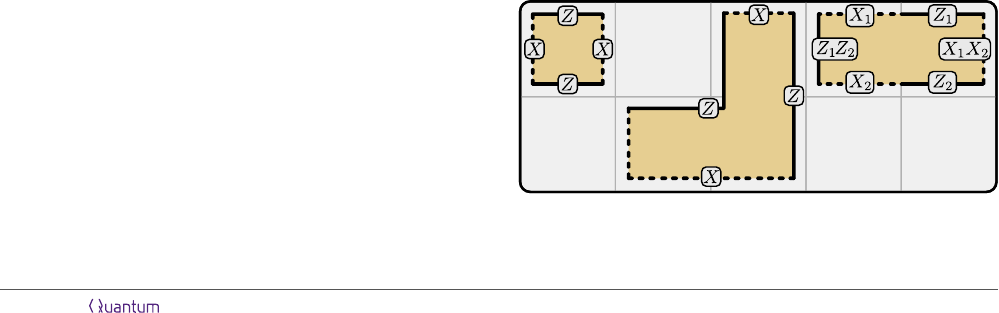A Game of Surface Codes:
Large-Scale Quantum Computing with Lattice Surgery
Daniel Litinski @ Dahlem Center for Complex Quantum Systems, Freie Universit
¨
at Berlin, Arnimallee 14, 14195 Berlin, Germany
Given a quantum gate circuit, how does one
execute it in a fault-tolerant architecture with
as little overhead as possible? In this pa-
per, we discuss strategies for surface-code quan-
tum computing on small, intermediate and large
scales. They are strategies for space-time trade-
oﬀs, going from slow computations using few
qubits to fast computations using many qubits.
Our schemes are based on surface-code patches,
which not only feature a low space cost com-
pared to other surface-code schemes, but are
also conceptually simple simple enough that
they can be described as a tile-based game with
a small set of rules. Therefore, no knowledge of
quantum error correction is necessary to under-
stand the schemes in this paper, but only the
concepts of qubits and measurements.
The ﬁeld of quantum computing is fuelled by the
promise of fast solutions to classically intractable prob-
lems, such as simulating large quantum systems or fac-
toring large numbers. Already 100 qubits can be used
to solve useful problems that are out of reach for clas-
sical computers [1, 2]. Despite the exponential speed-
up, the actual time required to solve these problems
is orders of magnitude above the coherence times of
any physical qubit. In order to store and manipulate
quantum information on large time scales, it is neces-
sary to actively correct errors by combining many phys-
ical qubits into logical qubits using a quantum error-
correcting code . Of particular interest are codes
that are compatible with the locality constraints of real-
istic devices such as superconducting qubits, which are
limited to operations that are local in two dimensions.
The most prominent such code is the surface code [6, 7].
Working with logical qubits introduces additional
overhead to the computation. Not only is the space cost
drastically increased as physical qubits are replaced by
logical qubits, but also the time cost increases due to
the restricted set of accessible logical operations. Sur-
face codes, in particular, are limited to a set of 2D-
local operations, which means that arbitrary gates in a
quantum circuit may require several time steps instead
of just one. To keep the cost of surface-code quan-
tum computing low, it is important to ﬁnd schemes
that translate quantum circuits into surface-code lay-
outs with a low space-time overhead. This is also nec-
essary to benchmark how well quantum algorithms per-
form in a surface-code architecture.
There exist several encoding schemes for surface
codes, among others, defect-based , twist-based 
and patch-based  encodings. In this work, we focus
on the latter. Surface-code patches have a low space
overhead compared to other schemes, and oﬀer low-
conceptually less diﬃcult to understand, as they do not
directly involve braiding of topological defects. Design-
ing computational schemes with surface-code patches
only requires the concepts of qubits and measurements.
To this end, we describe the operations of surface-code
patches as a tile-based game. This is helpful to design
protocols and determine their space-time cost. The ex-
act correspondence between this game and surface-code
patches is speciﬁed in Appendix A, but it is not crucial
for understanding this paper. Readers who are inter-
ested in the detailed surface-code operations may read
Appendix A in parallel to the following section.
Surface codes as a game. The game is played on
a board partitioned into a number of tiles. An example
of a 5 × 2 grid of tiles is shown in Fig. 1. The tiles
can be used to host patches, which are representations
of qubits. We denote the Pauli operators of each qubit
as X, Y and Z. Patches have dashed and solid edges
representing Pauli operators. We consider two types of
patches: one-qubit and two-qubit patches. One-qubit
patches represent one qubit and consist of two dashed
and two solid edges. Each of the two dashed (solid)
edges represent the qubit’s X (Z) operator. While the
square patch in Fig. 1a only occupies one tile, a one-
qubit patch can also be shaped to, e.g., occupy three
tiles (b). A two-qubit patch (c) consists of six edges and
represents two qubits. The ﬁrst qubit’s Pauli operators
X
1
and Z
1
are represented by the two top edges, while
(a)
(b)
(c)
Figure 1: Examples of one-qubit (a/b) and two-qubit (c)
patches in a 5 × 2 grid of tiles.
Accepted in Quantum 2019-02-01, click title to verify 1
arXiv:1808.02892v3 [quant-ph] 3 Feb 2019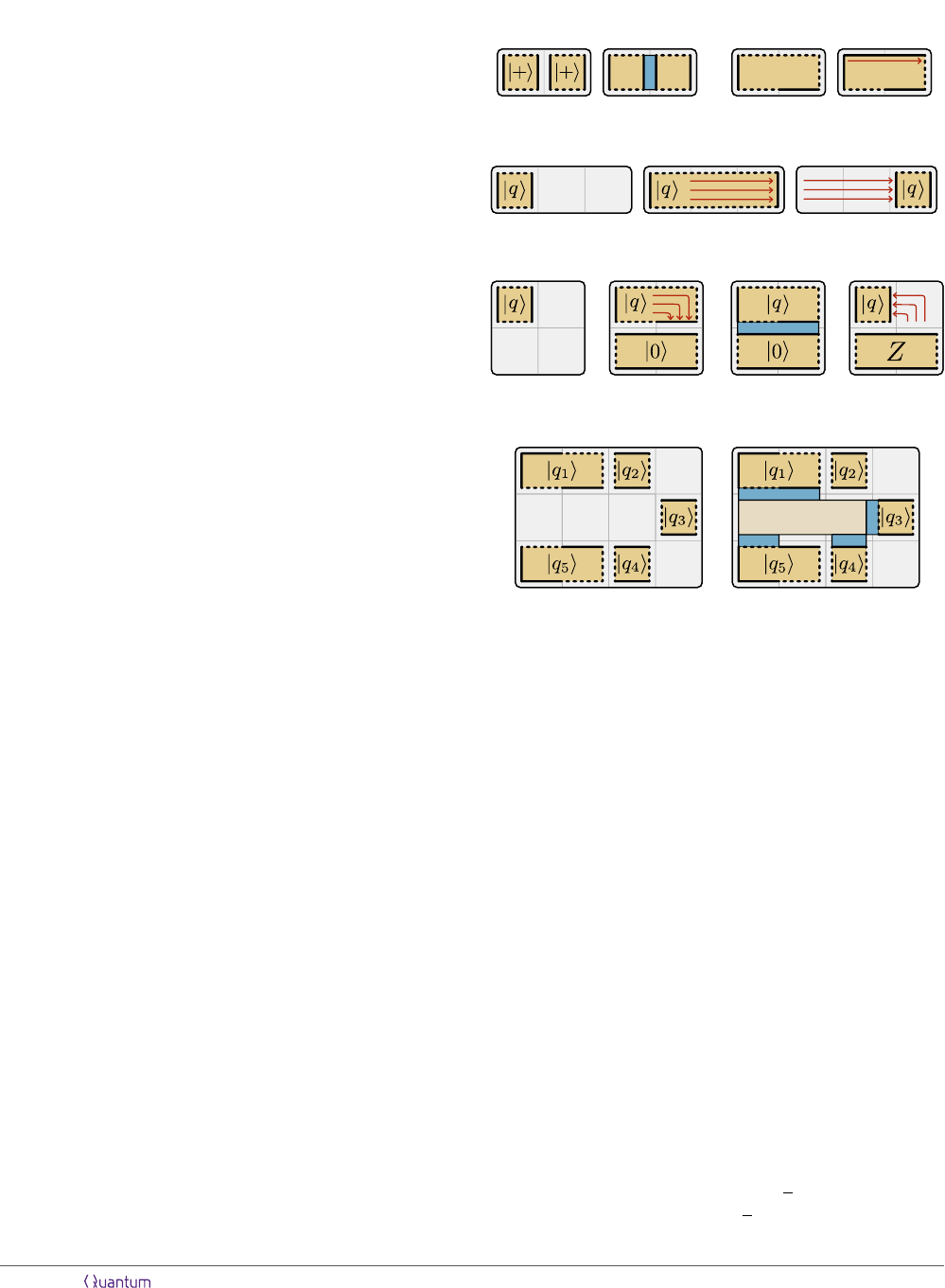the second qubit’s operators X
2
and Z
2
are found in the
two bottom edges. The remaining two edges represent
the operators Z
1
· Z
2
and X
1
· X
2
.
In the following, we specify the operations that can be
used to manipulate the qubits represented by patches.
Some of these operations take one time step to complete
(denoted by 1), whereas others can be performed in-
stantly, requiring 0. The goal is to implement quan-
tum algorithms using as few tiles and time steps as pos-
sible. There are three types of operations: qubit initial-
ization, qubit measurement and patch deformation.
I. Qubit initialization:
One-qubit patches can be initialized in the X
and Z eigenstates |+i and |0i. (Cost: 0)
Two-qubit patches can be initialized in the
states |+i |+i and |0i |0i. (Cost: 0)
One-qubit patches can be initialized in an ar-
bitrary state. Unless this state is |+i or |0i,
an undetected random Pauli error may spoil
the qubit with probability p. (Cost: 0)
II. Qubit measurement:
Single-patch measurements: The qubits rep-
resented by patches can be measured in the
X or Z basis. For two-qubit patches, the two
qubits must be measured simultaneously and
in the same basis. This measurement removes
the patch from the board, freeing up previ-
ously occupied tiles. (Cost: 0)
Two-patch measurements: If edges of two dif-
ferent patches are positioned in adjacent tiles,
the product of the operators of the two edges
can be measured. For example, the product
ZZ between two neighboring square patches
can be measured, as highlighted in step 2 of
Fig. 2a by the blue rectangle. If the edge of
one patch is adjacent to multiple edges of the
other patch, the product of all involved Pauli
operators can be measured. For instance, if
qubit A’s Z edge is adjacent to both qubit
B’s X edge and Z edge, the operator Z
A
Y
B
can be measured (see step 3 of Fig. 2d), since
Y = iXZ. (Cost: 1)
Multi-patch measurements: An arbitrarily-
shaped ancilla patch can be initialized. The
product of any number of operators adjacent
to the ancilla patch can be measured. The an-
cilla patch is discarded after the measurement.
The example of a Y
|q
1
i
X
|q
3
i
Z
|q
4
i
X
|q
5
i
measurement is shown in Fig. 2e. (Cost: 1)
0 Step 1 1 Step 2
0 Step 1
(c) Qubit movement
1 Step 2 1 Step 3
(d) Y basis measurement
0 Step 1 1 Step 2 2 Step 3 2 Step 4
0 Step 1 1 Step 2
(b) Moving corners(a) Bell state preparation
0 Step 1 1 Step 2
(e) Y
|q
1
i
X
|q
3
i
Z
|q
4
i
X
|q
5
i
measurement
ancilla
Figure 2: Examples of short protocols. (a) Preparation of a
two-qubit Bell state in 1. (b) Moving corners of a four-corner
patch to change its shape in 1. (c) Moving a square-patch
qubit over long distances in 1. (d) Measurement of a square-
patch qubit in the Y basis using an ancilla qubit and 2. (e) A
multi-qubit Y
|q
1
i
X
|q
3
i
Z
|q
4
i
X
|q
5
i
measurement in 1.
III. Patch deformation:
Edges of a patch can be moved to deform the
patch. If the edge is moved onto a free tile
to increase the size of the patch, this takes
1 to complete. If the edge is moved inside
the patch to make the patch smaller, the ac-
tion can be performed instantly.
Corners of a patch can be moved along the
patch boundary to change its shape, as shown
in Fig. 2b. (Cost: 1)
To illustrate these operations, we go through three
short example protocols in Fig. 2a/c/d. The ﬁrst ex-
ample (a) is the preparation of a Bell pair. Two square
patches are initialized in the |+i state. Next, the oper-
ator Z Z is measured. Before the measurement, the
qubits are in the state |+i|+i = (|00i+ |01i+ |10i+
|11i)/2. If the measurement outcome is +1, the qubits
end up in the state (|00i + |11i)/
2. For the outcome
1, the state is (|01i+|10i)/
2. In both cases, the two
Accepted in Quantum 2019-02-01, click title to verify 2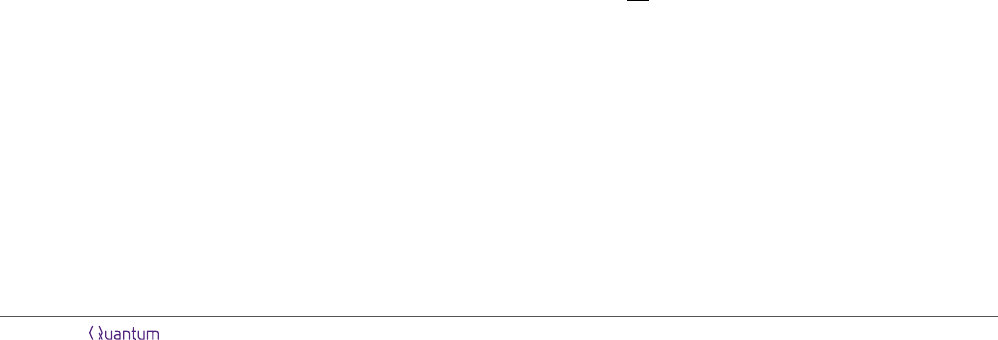qubits are in a maximally entangled Bell state. This
protocol takes 1 to complete. The second example (c)
is the movement of a square patch into a diﬀerent tile.
For this, the square patch is enlarged by patch defor-
mation, which takes 1, and then made smaller again
at no time cost. The third example (d) is the measure-
ment of a square patch in the Y basis. For this, the
patch is deformed such that the X and Z edge are on
the same side of the patch. An ancillary patch is ini-
tialized in the |0i state and the operator Z Y between
the ancilla and the qubit is measured. The ancilla is
discarded by measuring it in the Z basis.
Translation to surface codes. As described in
Appendix A, protocols designed within this framework
can be straightforwardly translated into surface-code
operations. Essentially, patches correspond to surface-
code patches with dashed and solid edges as rough and
smooth boundaries. Thus, for surface codes with a code
distance d, each tile corresponds to d
2
physical data
qubits. Each time step roughly corresponds to d code
cycles, i.e., measuring all surface-code check operators
d times. We associate a time step with all surface-code
operations which have a time cost that scales with d, but
no time step with operations whose time cost is inde-
pendent of the code distance, but may still be nonzero.
For this reason, the correspondence between 1 and d
code cycles is not exact.
Two-patch and multi-patch measurements corre-
spond to (twist-based) lattice surgery [9, 11] and multi-
qubit lattice surgery , respectively, which both re-
quire d code cycles to account for measurement errors.
Qubit initialization has no time cost, since, in the case
of X and Z eigenstates, it can be done simultaneously
with the subsequent lattice surgery [9, 13]. For arbi-
trary states, initialization corresponds to state injec-
tion [13, 14]. Its time cost does not scale with d. Simi-
larly, single-qubit measurements in the X or Z basis cor-
respond to the simultaneous measurement of all phys-
ical data qubits in the corresponding basis and some
classical error correction, which does not scale with d
either. Patch deformation is code deformation, which
requires d code cycles, unless the patch becomes smaller
in the process, in which case it corresponds to single-
qubit measurements. Note that not all surface-code op-
erations are covered by this framework. An extended
set of rules is discussed in Appendix B.
In essence, the framework can be used to estimate the
space-time cost of a computation. The leading-order
term of the space-time cost the term that scales with
d
3
of a protocol that uses s tiles for t time steps is
st · d
3
in terms of (physical data qubits)·(code cycles).
The space cost is s ·d
2
physical data qubits. Determin-
ing the exact time cost requires special care. In some
protocols, the subleading contributions due to state in-
jection and classical processing may need to be taken
into account. For these protocols, we will show how
they can be adapted to prevent such contributions from
increasing the time cost beyond t · d code cycles.
Overview
Having established the rules of the game and the corre-
spondence of our framework to surface-code operations,
our goal is to implement arbitrary quantum computa-
tions. In this work, we discuss strategies to tackle the
following problem: Given a quantum circuit, how does
one execute it as fast as possible on a surface-code-based
quantum computer of a certain size? This is an opti-
mization problem that was shown to be NP-hard , so
the focus is on heuristics rather than a general solution.
The content of this paper is outlined in Fig. 3.
The input to our problem is an arbitrary gate cir-
cuit corresponding to the computation. We refer to the
qubits that this circuit acts on as data qubits. As we
review in Sec. 1, the natural universal gate set for sur-
face codes is Cliﬀord+T , where Cliﬀord gates are cheap
and T gates are expensive. In fact, Cliﬀord gates can
be treated entirely classically, and T gates require the
consumption of a magic state |0i+e
/4
|1i. Only faulty
(undistilled ) magic states can be prepared in our frame-
work. To generate higher-ﬁdelity magic states for large-
scale quantum computation, a lengthy protocol called
magic state distillation  is used.
It is therefore natural to partition a quantum com-
puter into a block of tiles that is used to distill magic
states (a distillation block) and a block of tiles that
hosts the data qubits (a data block) and consumes
magic states. The speed of a quantum computer is gov-
erned by how fast magic states can be distilled, and how
fast they can be consumed by the data block.
In Sec. 2, we discuss how to design data blocks. In
particular, we show three designs: compact, intermedi-
ate and fast blocks. The compact block uses 1.5n + 3
tiles to store n qubits, but takes up to 9 to consume
a magic state. Intermediate blocks use 2n + 4 tiles and
require up to 5 per magic state. Finally, the fast block
uses 2n +
8n + 1 tiles, but requires only 1 to con-
sume a magic state. The compact block is an option for
early quantum computers with few qubits, where the
generation of a single magic state takes longer than 9.
The fast block has a better space-time overhead, which
makes it more favorable on larger scales.
Data blocks need to be combined with distillation
blocks for universal quantum computing. In Sec. 3,
we discuss designs of distillation blocks. Since magic
state distillation is the main operation of a surface-
code-based quantum computer, it is important to min-
imize its space-time cost. We discuss distillation proto-
Accepted in Quantum 2019-02-01, click title to verify 3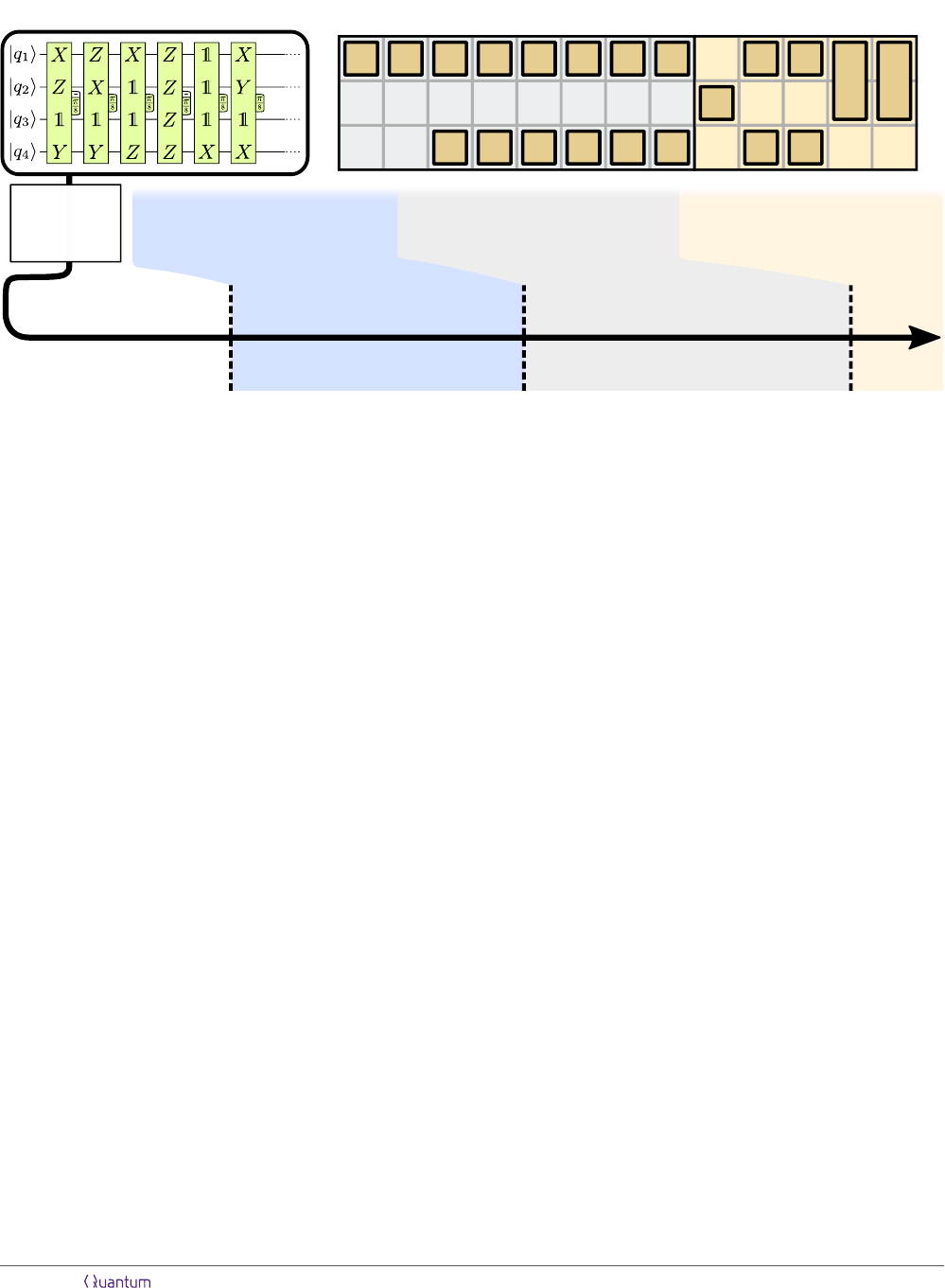Sec. 1: Cliﬀord+T circuits Sec. 2: Data blocks Sec. 3: Distillation blocks
Sec. 4:
55,000 qubits
4 hours
310,000 qubits
7 hours
120,000 qubits
22 minutes
1,000,000 qubits
45 minutes
1500 × 220,000 = 330m qubits
1 second
3000 × 1,500,000 4.5b qubits
1 second
p = 10
4
p = 10
3
d = 13
d = 27
Sec. 5:
Sec. 6:
Example:
100 qubits
10
8
T gates
···
···
···
···
100 qubits
(App endix C)
Figure 3: Overview of the content of this paper. To illustrate the space-time trade-oﬀs discussed in this work, we show the number
of physical qubits and the computational time required for a circuit of 10
8
T gates distributed over 10
6
T layers. We consider
physical error rates of p = 10
4
and p = 10
3
, for which we need code distances d = 13 and d = 27, respectively. We assume
that each code cycle takes 1 µs.
cols based on error-correcting codes with transversal T
gates, such as punctured Reed-Muller codes [16, 17] and
block codes . In comparison to braiding-based
implementations of distillation protocols, we reduce the
space-time cost by up to 90%.
A data block combined with a distillation block con-
stitutes a quantum computer in which T gates are per-
formed one after the other. At this stage, the quan-
tum computer can be sped up by increasing the num-
ber of distillation blocks, eﬀectively decreasing the time
it takes to distill a single magic state, as we discuss
in Sec. 4. In order to illustrate the resulting space-
time trade-oﬀ, we consider the example of a 100-qubit
computation with 10
8
T gates, which can already be
used to solve classically intractable problems . As-
suming an error rate of p = 10
4
and a code-cycle time
of 1 µs, a compact data block together with a distillation
block can ﬁnish the computation in 4 hours using 55,000
physical qubits.
1
Adding 10 more distillation blocks in-
creases the qubit count to 120,000 and decreases the
computational time to 22 minutes, using 1 per T gate.
For further space-time trade-oﬀs in Sec. 5, we exploit
that the T gates of a circuit are arranged in layers of
gates that can be executed simultaneously. This en-
ables linear space-time trade-oﬀs down to the execution
1
We will assume that the total number of physical qubits is
twice the number of physical data qubits. This is consistent with
superconducting qubit platforms, where the use of measurement
ancillas doubles the qubit count. If a platform does not require
the use of ancilla qubits, the total qubit count is reduced by 50%
compared to the numbers reported in this paper.
of one T layer per qubit measurement time, eﬀectively
implementing Fowler’s time-optimal scheme . If the
10
8
T gates are distributed over 10
6
layers, and mea-
surements (and classical processing) can be performed
in 1 µs, up to 1500 units of 220,000 qubits can be run in
parallel, where each unit is responsible for the execution
of one T layer. This way, the computational time can
be brought down to 1 second using 330 million qubits.
While this is a large number, the units do not necessar-
ily need to be part of the same quantum computer, but
can be distributed over up to 1500 quantum computers
with 220,000 qubits each, and with the ability to share
Bell pairs between neighboring computers.
In Sec. 6, we discuss further space-time trade-oﬀs that
are beyond the parallelization of Cliﬀord+T circuits. In
particular, we discuss the use of Cliﬀord+ϕ circuits, i.e.,
circuits containing arbitrary-angle rotations beyond T
gates. These require the use of additional resources,
but can speed up the computation. We also discuss the
possibility of hardware-based trade-oﬀs by using higher
code distances, but in turn shorter measurements with
a decreased measurement ﬁdelity. Ultimately, the speed
of a quantum computer is limited by classical process-
ing, which can only be improved upon by faster classical
computing.
Finally, we note that while the number of qubits re-
quired for useful quantum computing is orders of mag-
nitude above what is currently available, a proof-of-
principle two-qubit device demonstrating all necessary
operations using undistilled magic states can be built
with 48 physical data qubits, see Appendix C.
Accepted in Quantum 2019-02-01, click title to verify 4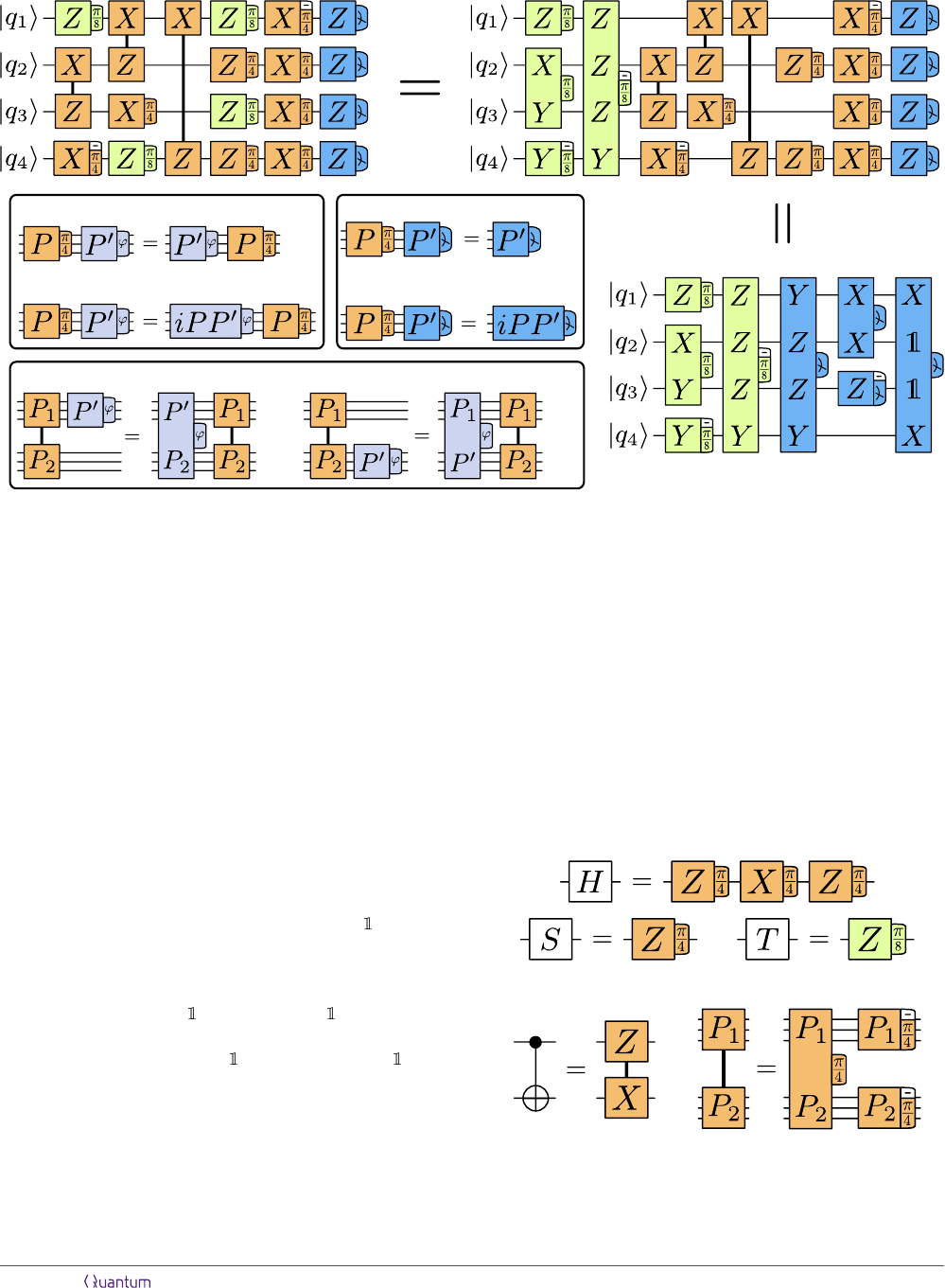if P P
0
= P
0
P :
if P P
0
= P
0
P :
(a)
if P
1
P
0
= P
0
P
1
: if P
2
P
0
= P
0
P
2
:
if P P
0
= P
0
P :
if P P
0
= P
0
P :
(c)
(b)
(a/b)
(c)
Figure 4: A generic circuit consists of π/4 rotations (orange), π/8 rotations (green) and measurements (blue). The Pauli product
in each box speciﬁes the axis of rotation or the basis of measurement. If the Pauli operator is P instead of P , a minus sign
is found in the corner of the box, such that, e.g., Z
π/4
corresponds to an S
gate. Using the commutation rules in (a/b), all
Cliﬀord gates can be moved to the end of the circuit. Using (c), the Cliﬀord gates can be absorbed by the ﬁnal measurements.
1 Cliﬀord+T quantum circuits
Our goal is to implement full quantum algorithms with
surface codes. The input to our problem is the al-
gorithm’s quantum circuit. The universal gate set
Cliﬀord+T is well-suited for surface codes, since it sepa-
rates easy operations from diﬃcult ones. Often, this set
is generated using the Hadamard gate H, phase gate S,
controlled-NOT (CNOT) gate, and the T gate. Instead,
we choose to write our circuits using Pauli product ro-
tations P
ϕ
(see Fig. 5), because it simpliﬁes circuit ma-
nipulations. Here, P
ϕ
= exp(iP ϕ), where P is a Pauli
product operator (such as Z, Y X, or X X) and
ϕ is an angle. In this sense, S = Z
π/4
, T = Z
π/8
,
and H = Z
π/4
· X
π/4
· Z
π/4
. The CNOT gate can
also be written in terms of Pauli product rotations as
CNOT = (Z X)
π/4
·( X)
π/4
·(Z )
π/4
. In fact,
we can more generally deﬁne P
1
-controlled-P
2
gates as
C(P
1
, P
2
) = (P
1
P
2
)
π/4
· ( P
2
)
π/4
· (P
1
)
π/4
.
The CNOT gate is the speciﬁc case of C(Z, X).
Getting rid of Cliﬀord gates. Cliﬀord gates are
considered to be easy, because, by deﬁnition, they map
Pauli operators onto other Pauli operators . This
can be used to simplify the input circuit. A generic cir-
cuit is shown in Fig. 4, consisting of Cliﬀord gates, Z
π/8
rotations and Z measurements. If all Cliﬀord gates are
commuted to the end of the circuit, the Z
π/8
rotations
become Pauli product rotations. The rules for moving
P
π/4
rotations past P
0
ϕ
gates are shown in Fig. 4a: If P
and P
0
commute, P
π/4
can simply be moved past P
0
ϕ
.
If they anticommute, P
0
ϕ
turns into (iP P
0
)
ϕ
when P
π/4
is moved to the right. Since C(P
1
, P
2
) gates consist
of π/4 rotations, similar rules can be derived as shown
(a) Single-qubit rotations
(b) CNOT (c) C(P
1
, P
2
) gate
Figure 5: Cliﬀord+T gates in terms of Pauli rotations.
(a) Single-qubit Cliﬀord gates are π/4 rotations, and the T
gate is a π/8 rotation. (b/c) P
1
-controlled-P
2
gates are Clif-
ford gates, where C(Z, X) is the CNOT gate.
Accepted in Quantum 2019-02-01, click title to verify 5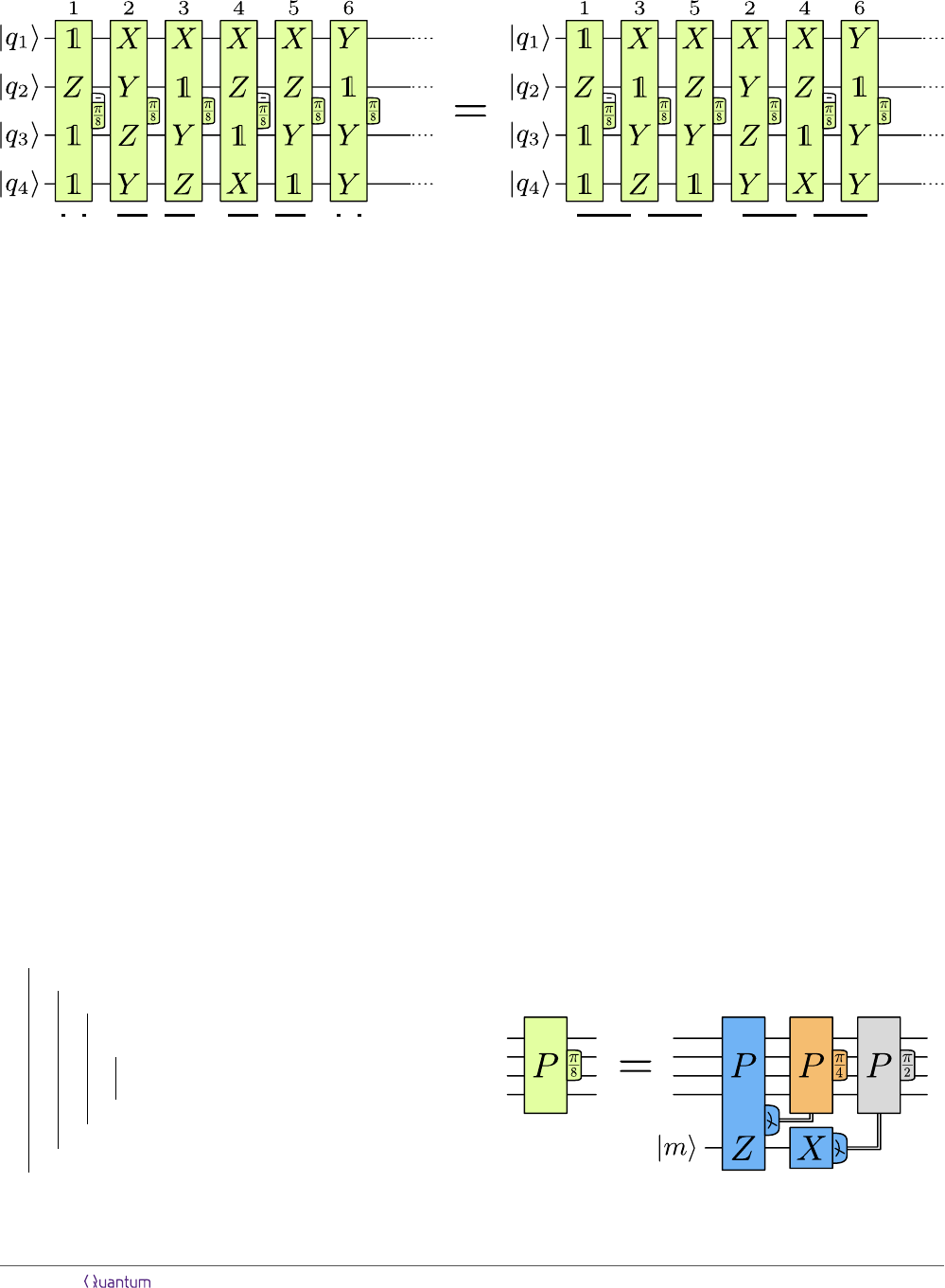| {z }
layer 2
|{z}
layer 1
| {z }
layer 3
|{z}
layer 4
| {z }
layer 1
| {z }
layer 2
Figure 6: Cliﬀord+T circuits can be written as a number of consecutive π/8 rotations. These gates are grouped into layers of
mutually commuting rotations. A simple greedy algorithm can be used to reduce the number of layers, i.e., the T depth.
in Fig. 4b: If P
0
anticommutes with P
1
, P
0
ϕ
turns into
(P
0
P
2
)
ϕ
after commutation. If P
0
anticommutes with
P
2
, P
0
ϕ
turns into (P
0
P
1
)
ϕ
. If P
0
anticommutes with
both P
1
and P
2
, P
0
ϕ
turns into (P
0
P
1
P
2
)
ϕ
.
After moving the Cliﬀord gates to the right, the re-
sulting circuit consists of three parts: a set of π/8 ro-
tations, a set of π/4 rotations, and Z measurements.
Because Cliﬀord gates map Pauli operators onto other
Pauli operators, the Cliﬀord gates can be absorbed by
the ﬁnal measurements, turning Z measurements into
Pauli product measurements. The commutation rules
of this ﬁnal step are shown in Fig. 4c and are similar to
the commutation of Cliﬀord gates past rotations.
T count and T depth. Thus, every n-qubit circuit
can be written as a number of consecutive π/8 rotations
and n ﬁnal Pauli product measurements, as shown in
Fig. 6. We refer to the number of π/8 rotations as the
T count. An important part of circuit optimization is
the minimization of the T count, for which there ex-
ist various approaches . The π/8 rotations of
a circuit can be grouped into layers. All π/8 rotations
that are part of a layer need to mutually commute. The
number of π/8 layers of a circuit is strictly speaking not
the same quantity as the T depth, but we will still refer
to it as the T depth and to π/8 layers as T layers. Note
repeat
for each layer i do
for each rotation j in layer i + 1 do
if (rotation j commutes with all
rotations in layer i) then
Move rotation j from layer i + 1 to
layer i;
end
end
end
until the partitioning no longer changes;
Algorithm to reduce the T count and T depth.
that, in the usual deﬁnition, only up to n T gates can
be part of a layer, whereas in our case, there is no limit.
When partitioning π/8 rotations into layers, the naive
approach often yields more layers than are necessary.
For instance, a naive partitioning of the ﬁrst 6 T gates
of Fig. 6 yields 4 layers. A few commutations can bring
the number down to 2 layers. There are a number of
algorithms for the optimization of the T depth .
Here, we use the simple greedy algorithm shown below
to reduce the number of layers.
Note that when a reordering puts two equal π/8 rota-
tions into the same layer, they can be combined into a
π/4 rotation that is commuted to the end of the circuit,
thereby decreasing the T count. As we discuss in Sec. 6,
this kind of algorithm can not only be used with π/8 ro-
tations, but, in principle, with arbitrary Pauli product
rotations. The reduction of the circuit depth in terms
of non-π/8 rotations can be useful when going beyond
Cliﬀord+T circuits.
1.1 Pauli product measurements
When implementing circuits like Fig. 6 with surface
codes, one obstacle is that π/8 rotations are not di-
rectly part of the set of available operations. Instead,
one uses magic states  as a resource. These states
are π/8-rotated Pauli eigenstates |mi = |0i + e
/4
|1i.
They can be consumed in order to perform P
π/8
rota-
tions. The corresponding circuit  is shown in Fig. 7.
Figure 7: Circuit to perform a π/8 rotation by consuming a
magic state.
Accepted in Quantum 2019-02-01, click title to verify 6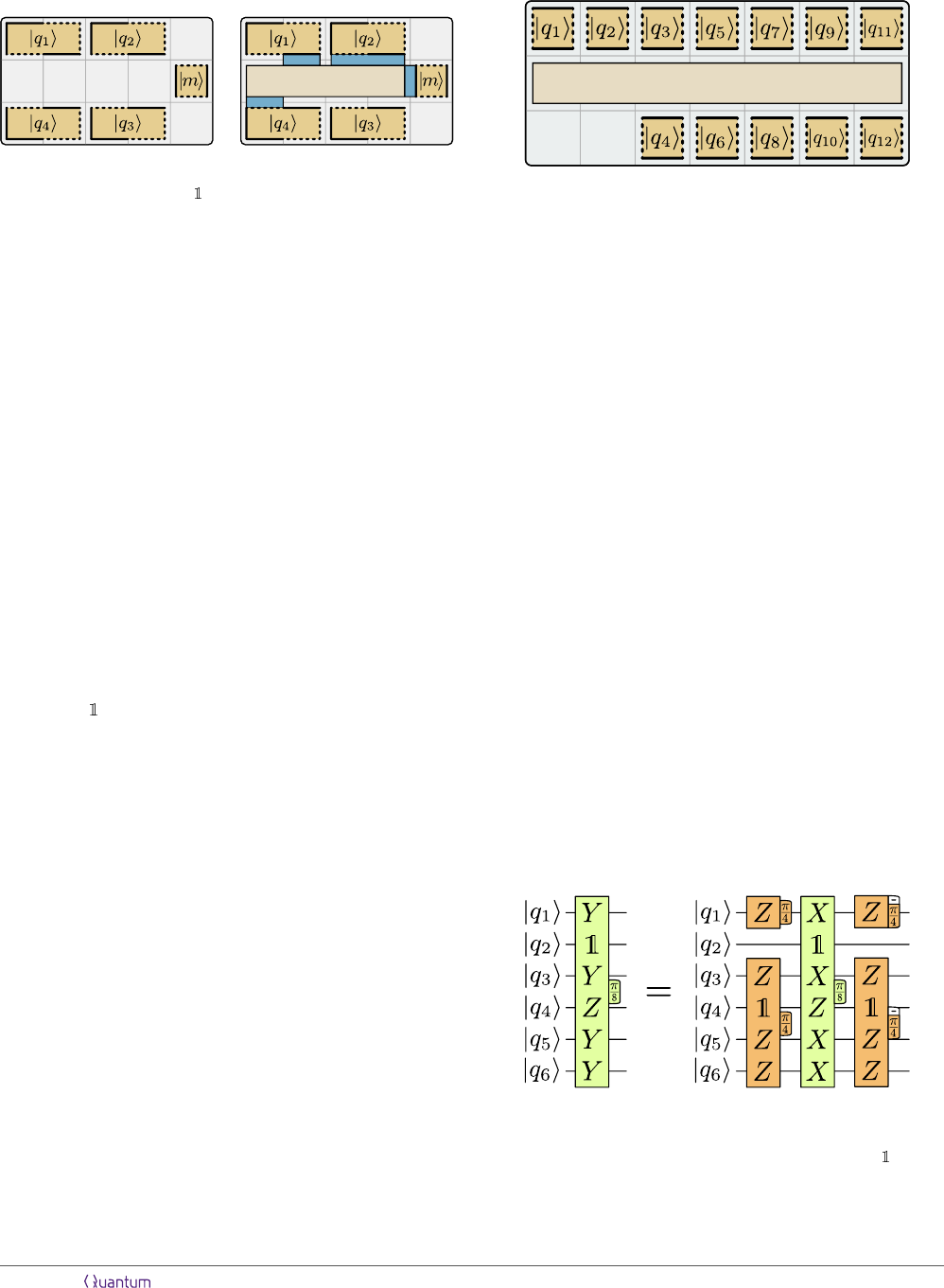0 Step 1 1 Step 2
Figure 8: Example of a Z
|q
1
i
Y
|q
2
i
X
|q
4
i
Z
|mi
measurement
to implement a (Z Y X)
π/8
gate.
A P
π/8
rotation corresponds to a P Z measurement
involving the magic state. If the measurement outcome
is P Z = 1, then a corrective P
π/4
operation is
necessary. Since this is a Cliﬀord gate, it can be sim-
ply commuted to the end of the circuit, changing the
axes of the subsequent π/8 rotations. Finally, in or-
der to discard the magic state, it is disentangled from
the rest of the system by an X measurement. Here,
an outcome X = 1 prompts a P
π/2
correction. π/2
rotations correspond to Pauli operators, i.e., P
π/2
= P .
The Pauli correction can also be commuted to the end
of the circuit. When P
π/2
is moved past a P
0
rotation
or measurement, it changes the axis of rotation or mea-
surement basis to P
0
, if P and P
0
anticommute.
In essence, if magic states are available, the only
operations required for universal quantum computing
are Pauli product measurements. In our framework,
such operations can be performed in 1 via multi-
patch measurements, corresponding to multi-qubit lat-
tice surgery. An example is shown in Fig. 8, where a
(Z Y X)
π/8
rotation on four qubits |q
1
i-|q
4
i
stored in four two-tile one-qubit patches is performed.
Using the circuit identity in Fig. 7, this is done by mea-
suring Z
|q
1
i
Y
|q
2
i
X
|q
4
i
Z
|mi
between the four qubits
and a magic state.
Summary. Cliﬀord+T circuits can be written in
terms of π/8 rotations, π/4 rotations and measure-
ments. To convert input circuits into a standard form,
π/4 rotations can be commuted to the end of the cir-
cuit and absorbed by the ﬁnal measurements. Thus, any
quantum computation can be written as a sequence of
π/8 rotations grouped into layers of mutually commut-
ing rotations. The number of rotations is the T count
and the number of layers is the T depth. Each rotation
can be performed by consuming a magic state via a
Pauli product measurement. These measurements can
be implemented in our framework in 1.
2 Data blocks
Since Cliﬀord+T circuits are a sequence of π/8 rota-
tions, each requiring the consumption of a magic state,
it is natural to partition a quantum computer into a set
ancilla region
Figure 9: A compact block stores n data qubits in 1.5n + 3
tiles. The consumption of a magic state can take up to 9.
of tiles that are used for magic state distillation (distil-
lation blocks) and a set of tiles that host data qubits and
consume magic states via Pauli product measurements
(data blocks). In this section, we discuss designs for
the latter. In principle, the structure shown in Fig. 8
is a data block, where each qubit is stored in a two-
tile patch and magic states can be consumed every 1.
However, this sort of design uses 3n tiles to host n data
qubits, which is a relatively large space overhead.
2.1 Compact block
The ﬁrst design that we discuss uses only 1.5n + 3 tiles.
This compact block is shown in Fig. 9, where each data
qubit is stored in a square patch. This lowers the space
cost, but restricts the operators that are accessible by
Pauli product measurements, as only the Z operator is
free to be measured. Using 3, patches may also be ro-
tated (see Fig. 11a), such that the X operator becomes
accessible instead of the Z operator. The problematic
operators are Y operators, which are the reason why
the consumption of a magic state can take up to 9.
The worst-case scenario is a π/8 rotation involv-
ing an even number of Y operators, such as the one
shown in Fig. 10. One possibility to replace Y oper-
ators by X or Z operators is via π/4 rotations, since
Figure 10: For compact blocks, the worst-case scenario are
Pauli product measurements involving an even number of Y
operators, e.g., the measurement required for a (Y ⊗ ⊗ Y
Z Y Y )
π/8
gate. Such measurements require two explicit
π/4 rotations (left), and two π/4 rotations that are commuted
to the end of the circuit (right).
Accepted in Quantum 2019-02-01, click title to verify 7